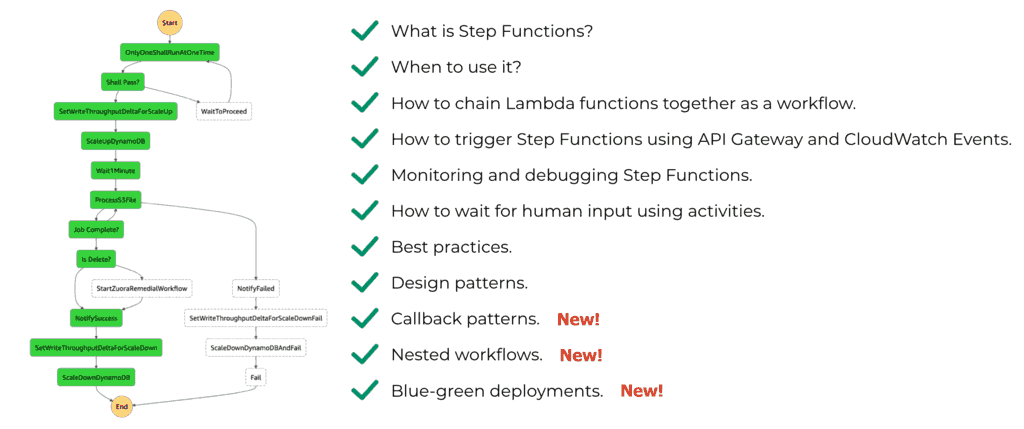# Project Euler – Problem 77 Solution

#### Problem

It is possible to write ten as the sum of primes in exactly five different ways:

7 + 3

5 + 5

5 + 3 + 2

3 + 3 + 2 + 2

2 + 2 + 2 + 2 + 2

What is the first value which can be written as the sum of primes in over five thousand different ways?

#### Solution

```// generate all prime numbers under <= this max
let max = 1000

// only check the prime numbers which are <= the square root of the number n
let hasDivisor n =
|> Seq.takeWhile (fun n' -> n' <= int(sqrt(double(n))))
|> Seq.exists (fun n' -> n % n' = 0)

// only check odd numbers <= max
let potentialPrimes = Seq.unfold (fun n -> if n > max then None else Some(n, n+2)) 3

// populate the prime numbers list
for n in potentialPrimes do if not(hasDivisor n) then primeNumbers <- primeNumbers @ &#91;n&#93;
let isPrime n = if n = 1 then false else not(hasDivisor(n))

// implement the coin change algorithm
let rec count n m (coins:int list) =
if n = 0 then 1
else if n < 0 then 0
else if (m <= 0 && n >= 1) then 0
else (count n (m-1) coins) + (count (n-coins.[m-1]) m coins)

let tuple =
Seq.unfold (fun state -> Some(state, state+1)) 10
|> Seq.map (fun n -> (n, primeNumbers |> Seq.filter (fun n' -> n' < n) |> Seq.cache))
|> Seq.filter (fun (n, l) -> count n (Seq.length l) (Seq.toList l) > 5000)

fst tuple
```

Yet another twist to problem 31 and problem 76, this time the coin change algorithm will be supplied with only prime numbers less than n.Hi, I’m Yan. I’m an AWS Serverless Hero and the author of Production-Ready Serverless.

I specialise in rapidly transitioning teams to serverless and building production-ready services on AWS.

Are you struggling with serverless or need guidance on best practices? Do you want someone to review your architecture and help you avoid costly mistakes down the line? Whatever the case, I’m here to help.Check out my new course, Complete Guide to AWS Step Functions. In this course, we’ll cover everything you need to know to use AWS Step Functions service effectively. Including basic concepts, HTTP and event triggers, activities, callbacks, nested workflows, design patterns and best practices.